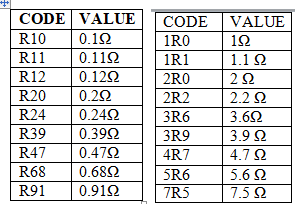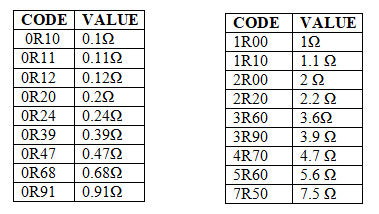## SMD-Resistor | Resistance code | Tolerance

### SMD Resistor

Surface Mount Device (SMD) it is often called a chip resistor these are in very small in size( dimensions) available in square or rectangular packages and mostly we used in PCB mounted device, it is designed to use with SMT (Surface Mount Technology). SMT is developed to achieve small area, high performance, and cheaper Unlike Through-Hole-Technology (THT) resistor devices, SMD resistors don’t have leads. Its resistance depends on the length, width, and material of a device to determine the power rating. Like normal resistor, these also have fixed-resistors and variable resistors (Trimmable Chip Resistor)

### Tolerance of  Resistor

At the time of the manufacturing process the resistance value may change a little bit, it may be deficiency or excess takes place in its value,
Example: 10kR ± 0.1
Here the resistance value is 10kΩ, but the manufacturing process the resistance value may be fixed at  10kΩ -0,1 or 10kΩ + 0.1,
Here 0.1 value is the tolerance value.

### What is the need of SMD Resistor

In our daily life technology will increases enormously, at the same time devices size had shown a high impact on manufactures as well as marketing area.
SMD Resistors are invented to there is a need for electronic devices to reduce their size as much as possible, corresponding to this we can reduce the circuit size then we can design a product with a compatible size.
Example: Mobile phones

### Factors affect the Miniaturization Technology

•      Technology will increases
•      Power will become decreases
•      The size of the device will reduce as per the user requirement
•      To achieve the better efficiency

### Types of SMD Resistors

Thermistor is the type of Resistor, the resistance of Thermistor depends on the temperature, these are classified into two types NTC (Negative Temperature coefficient), PTC (Positive temperature coefficient).

LDR  is the type of Resistor, here the resistance will depend on the Light intensity. When the light intensity are increases the resistance of the device will start to decrease when the light intensity will decrease the resistance of the device will starts decreases or does not change at a particular value.

LDR-Light dependant Resistor

Network Resistor  is the combination of more than one resistors is called Network resistor, they are available in a single package.

### How to check the resistance using Multimeter

Set the Multimeter red wire is connect at voltage position and Black wire is connected at the Ground (GND) of the multimeter. Now Consider your SMD-Resistor the value will be 100kΩ,  place the multimeter knob at the range of 10kΩ in a multimeter and connect the multimeter probes led pins to terminals of the resistor, then multimeter shows the resistance value in the display, maybe if it has shown ‘1’  number  it means that resistance is out of the range, you need to change the resistance range in the multimeter.

Note: SMD Resistor has no polarities you can connect in any terminals.

## Due to the small size, there is no chance to design color-code for calculating resistance value, so it is performed by “ code”  using numbers or alphabets. The most used codes are

Ø Three-digit code

Ø  Four digit code

Ø  Electronic Industrial Alliances (EIA)

#### Three-Digit code:

Its resistance value is like normal resistance calculation, the first two digits represent the significant values of the resistances and the third digit represents the multiplier. Multiplier means the power of ten which is multiplied with resistance value.

Standard resistor values: 105, 100, 223

## 100 stands for – 10 x 10^0 =100 Ω

223 stands for – 22 x 10^3 =22 KΩ

Other three-digit formats: R10, R47, R12, R68Resistor-color-code

### Four-digit code:

Its format is similar to the Three-Digit code, here the first three digits represent the significant values of the resistances and the fourth digit represents the multiplier. Multiplier means the power of ten which is multiplied with resistance value.

Standard resistor values: 7992, 2572, 1501

### Other four-digit format: 0R10 ,0R11,0R12,0R23,4R30Four-Digit  SMD-resistance

## Trimmable SMD-Resistors:

It is well known as variable resistors type, these are replaced the manual selector potentiometers these very use full in any adjustments (current /voltage) in design part. The resistance is particularly designed to allow flexibility with laser trimming process technologyLaser light is used to trim the resistance, so these are widely used in digital calibration designs.

#### PCB mounted circuit designPCB mounted SMD Resistors